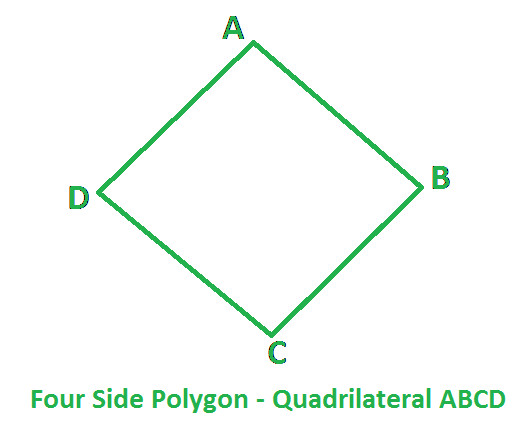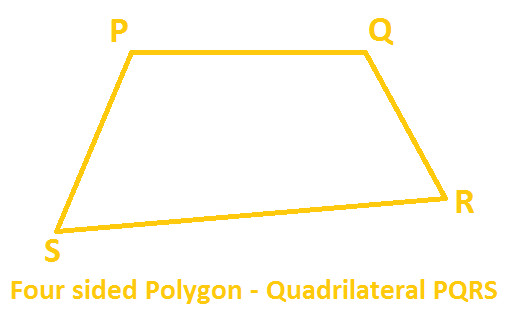Home >> Quadrilateral >>

Four Sided Polygon - Quadrilateral

Angle sum property of Quadrilateral Construction of Quadrilateral (compass)

What is a Polygon ?

"Quad" means Four, so a polygon which have 4 Angles, 4 Sides and 4 Vertices is known as Quadrilateral.In the Above diagram :-
4 Sides
- AB, BC, CD and DA
4 Angles - ABC, BCD, CDA and DAB
4 Vertices - A, B, C and DIn the Above diagram :-